# Chaos research today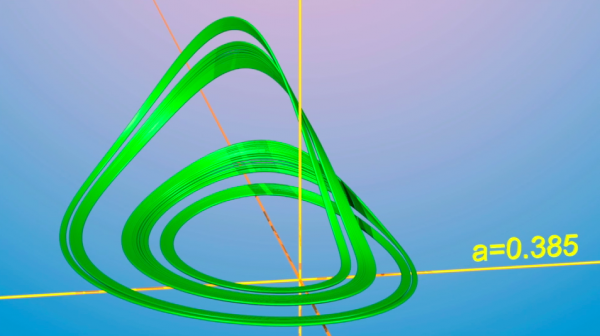There are many kinds of dynamics. Some are complicated, others are not. To try and understand this better, we can take a vector field that depends on just one parameter, and let this parameter change slowly. This shows that the dynamics, under influence of this parameter, is sometimes simple and then, without warning, becomes very complicated. We see bifurcations happening.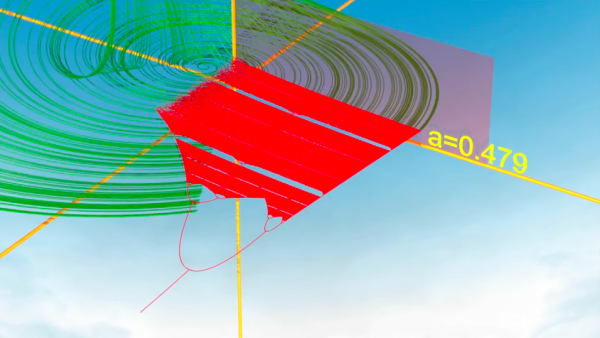These represent nice problems for mathematicians. The trace left by an attractor on a plane, when a parameter changes, can look like a piece of lace, and is called a bifurcation diagram.Mathematicians generally look for results that are always valid. However, more often than not, they start off with simple examples, hoping that the results they obtain are valid in general.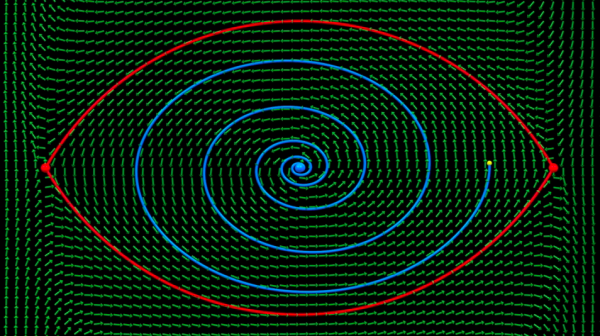On the Lorenz attractor, the proportion of time that a trajectory spends in a certain region, converges to a limit value over time. There is an SRB measure. Is it reasonable to expect that this is always the case? Unfortunately, the answer is no. This can be shown on a simple, but quite peculiar example discovered by Rufus Bowen (1947-1978). But the film shows that it is too early to leave this idea, and that in fact Bowen's example is very peculiar.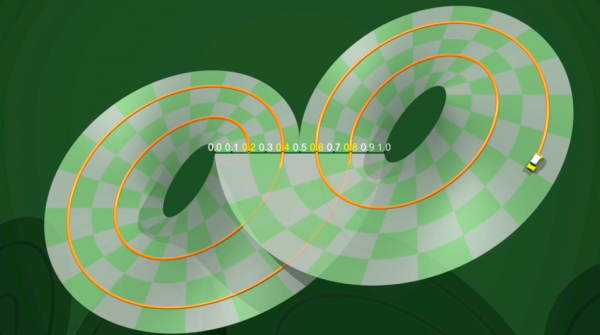Brasilian mathematician  Jacob Palis (1940-...), in the 1990's, formulated a set of problems that, if resolved, would permit a global view on chaos. Palis' conjectures are precise mathematical statements, and therefore rather technical, but we have run into some of them in our film:

• a typical vector field should only have a finite number of attractors ;
• an typical initial condition should be attracted by one of these attractors;
• to each attractor should be associated an SRB measure describing the asymptotic statistics of a typical trajectory attracted by this attractor;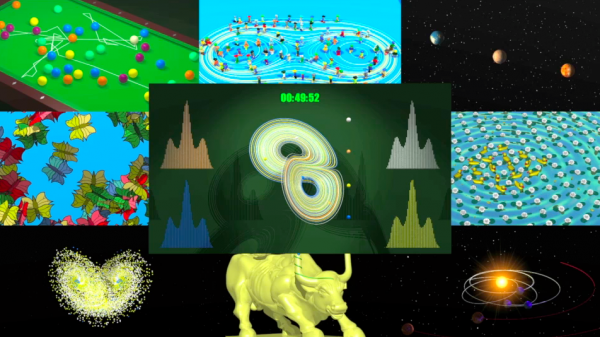Today we no longer think of determinism as the evolution of an individual trajectory, but rather as the collective evolution of a whole set. Sensitivity of trajectories to initial conditions is compensated by a kind of statistical stability of the whole set.

A whole group of mathematicians is hard at work on these conjectures, and they are being solved methodically,  bit by bit. A global picture seems to be emerging. Is this picture is too optimistic? Time will tell.